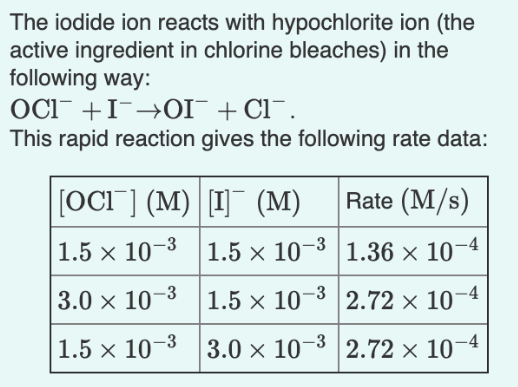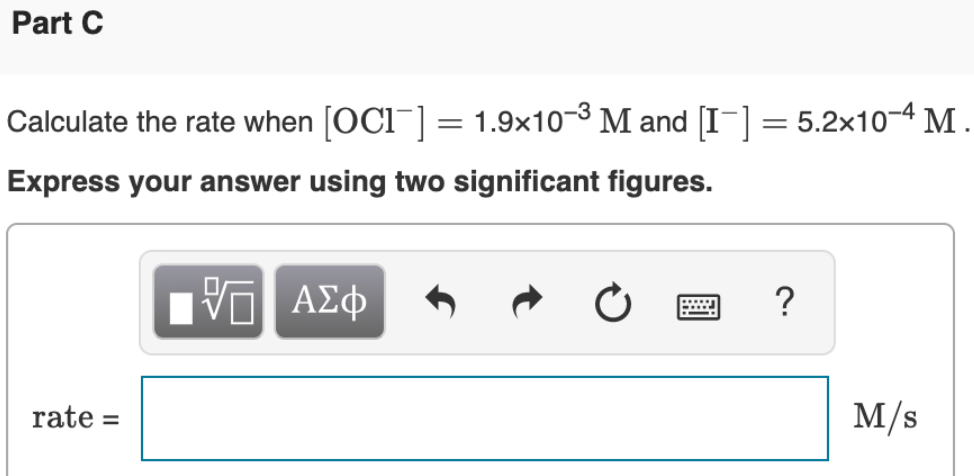# The iodide ion reacts with hypochlorite ion (the active ingredient in chlorine bleaches) in the following way: OCl^-+ I^- → OI^- + Cl^-. The rapid reaction gives the following rate data: Calculate the rate when [OCl^-] = 1.9x10^-3 M and [I^-] = 5.2x10^-4 M.